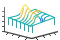# waterfall

•## 语法

``waterfall(X,Y,Z)``
``waterfall(X,Y,Z,C)``
``waterfall(Z)``
``waterfall(Z,C)``
``waterfall(ax,___)``
``p = waterfall(___)``

## 说明

``waterfall(X,Y,Z)` 创建瀑布图，这是一种沿 y 维度有部分帷幕的网格图。这会产生一种“瀑布”效果。该函数将矩阵 `Z` 中的值绘制为由 `X` 和 `Y` 定义的 x-y 平面中的网格上方的高度。边颜色因 `Z` 指定的高度而异。`

``waterfall(X,Y,Z,C)` 进一步指定边的颜色。`
``waterfall(Z)` 创建一个瀑布图，并将 `Z` 中元素的列索引和行索引用作 x 坐标和 y 坐标。`
``waterfall(Z,C)` 进一步指定边的颜色。`
``waterfall(ax,___)` 将图形绘制到 `ax` 指定的坐标区中，而不是当前坐标区中。指定坐标区作为第一个输入参数。此参数可用于上述任一输入语法。`

``p = waterfall(___)` 返回补片对象。在创建瀑布图后，使用 `p` 对其进行修改。有关属性列表，请参阅 Patch 属性。`

## 示例

```[X,Y] = meshgrid(-3:.125:3); Z = peaks(X,Y); waterfall(X,Y,Z)``````[X,Y] = meshgrid(-3:.125:3); Z = peaks(X,Y); C = gradient(Z); waterfall(X,Y,Z,C) colorbar``````[X,Y] = meshgrid(-5:.5:5); Z = Y.*sin(X) - X.*cos(Y); p = waterfall(X,Y,Z)``````p = Patch with properties: FaceColor: [1 1 1] FaceAlpha: 1 EdgeColor: 'flat' LineStyle: '-' Faces: [21x26 double] Vertices: [546x3 double] Show all properties ```

`p.EdgeColor = 'b';````[X,Y] = meshgrid(-3:.125:3); Z = peaks(X,Y); waterfall(X',Y',Z')```## 输入参数

x 坐标，指定为大小与 `Z` 相同的矩阵或长度为 n 的向量（当 `Z` 是 m×n 矩阵时）。如果未指定 `X``Y` 的值，`waterfall` 将使用向量 `(1:n)``(1:m)`

y 坐标，指定为大小与 `Z` 相同的矩阵或长度为 m 的向量（当 `Z` 是 m×n 矩阵时）。如果未指定 `X``Y` 的值，`waterfall` 将使用向量 `(1:n)``(1:m)`

z 坐标，指定为矩阵。`Z` 必须有至少两行两列。

`Z` 指定在每个 xy 坐标处的瀑布图的高度。如果不指定颜色，则 `Z` 还用于指定图边颜色。

## 提示

• 要以列（而不是行）形式分析数据，请调用带转置参数的 `waterfall`

```[X,Y] = meshgrid(-3:.125:3); Z = peaks(X,Y); waterfall(X',Y',Z')```

• 要创建网格曲面对象而不是补片对象，请使用 `meshz` 函数。要创建类似瀑布图的图，请将曲面的 `MeshStyle` 属性设置为 `'Row'`

## 算法

• 坐标区的 `XLim``YLim``ZLim` 属性存储 x、y 和 z 轴的范围。这些范围基于 `X``Y``Z` 输入参数的范围。

• 坐标区的 `CLim` 属性确定颜色在 `C` 范围内的分布。有关详细信息，请参阅控制颜色图范围